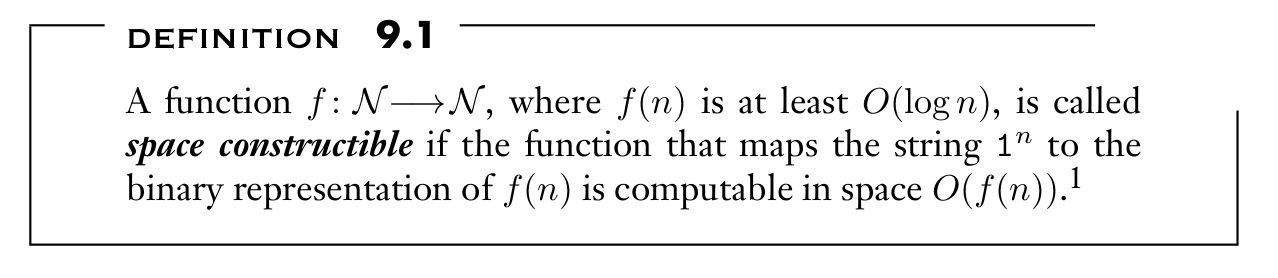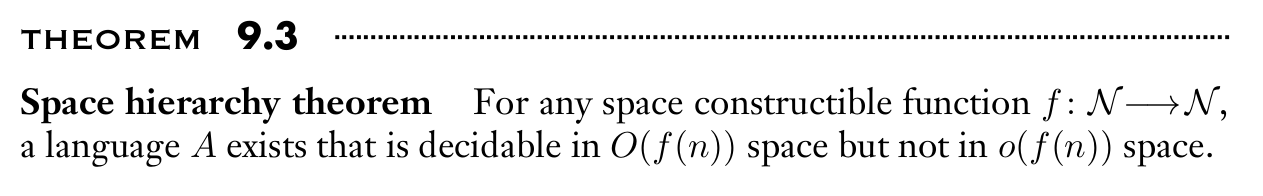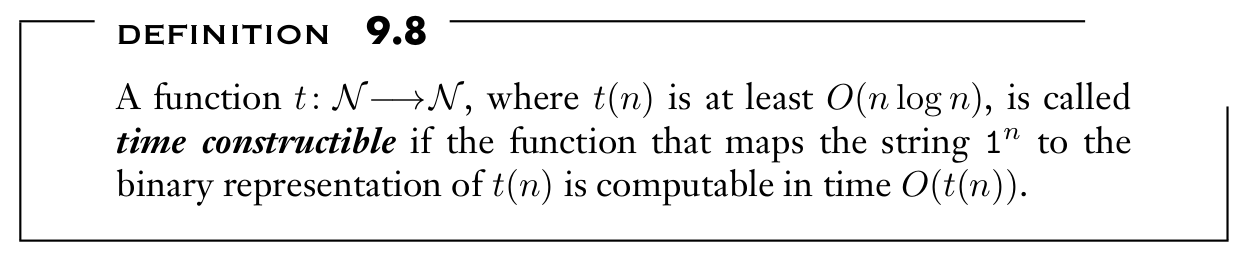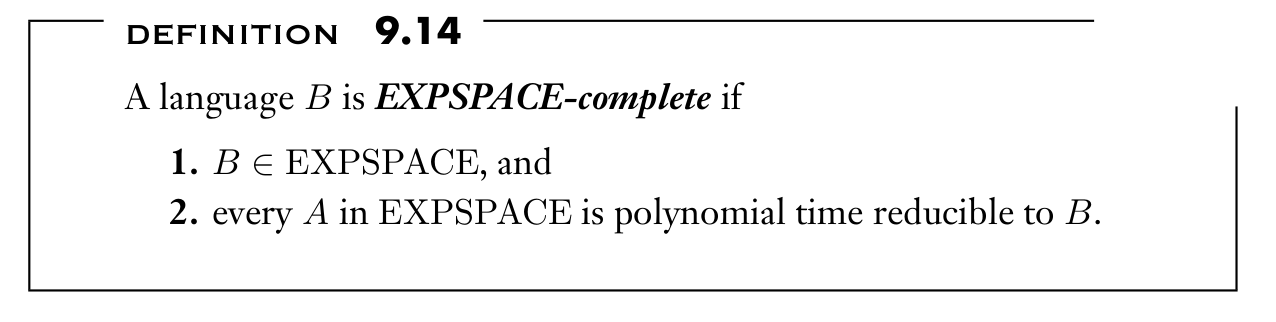## [ITOC-ch9] INTRACTABILITY

intractable

• Problems that are solvable in principle, but the solutions require so much time or space that they can’t be used in practice.

### 9.1 HIERARCHY THEOREMS

giving a Turing machine more time or more space should increase the class of problems that it can solve.

space constructible

• Example: All commonly occurring functions that are at least $O(log n)$ are space constructible, including the functions $log_2 n$ , $n log_2 n$, and $n^2$ .Space hierarchy theoremCOROLLARY 9.4

• For any two functions $f_1 , f_2 : N \longrightarrow N$, where $f_1 (n)$ is $o(f_2 (n))$ and $f_2$ is space constructible

$SPACE(f_1 (n)) \subsetneq SPACE(f_2 (n))$

COROLLARY 9.5

• For any two real numbers $0 ≤ \epsilon_1 < \epsilon_2$ , $SPACE(n^{\epsilon_1} ) \subsetneq SPACE(n^{\epsilon_2} )$.

COROLLARY 9.6

• $NL \subsetneq PSPACE$.
• $TQBF \in PSPACE$-complete, so $TQBF \notin NL$

COROLLARY 9.7

• $PSPACE \subsetneq EXPSPACE$
• establishes the existence of decidable problems that are intractable.

time constructible

• Example: All commonly occurring functions that are at least $n log n$ are time constructible, including the functions $n log n$, $n \sqrt n$, $n^2$ , and $2^n$Time hierarchy theoremCOROLLARY 9.11

• For any two functions $t_1 , t_2 : N \longrightarrow N$ , where $t_1 (n)$ is $o(t_2 (n)/ logt_2 (n))$ and $t_2$ is time constructible,

$TIME(t_1 (n)) \subsetneq TIME(t_2 (n))$

COROLLARY 9.12

• For any two real numbers $1 ≤ \epsilon_1 < \epsilon_2$ , $TIME(n^{\epsilon_1} ) \subsetneq TIME(n^{\epsilon_2} )$

COROLLARY 9.13

• $P \subsetneq EXPTIME$

EXPONENTIAL SPACE COMPLETENESS

demonstrate that a specific language intractable:

• TM can decide more languages in EXPSPACE than it can in PSPACE
• show that the language is complete for EXPSPACE

EXPSPACE-complete$EQ_{REX↑} = \{ < Q, R > | \ Q \ and \ R \ are \ equivalent \ regular \ expressions \ with \ exponentiation\}.$

Proof

• $EQ_{REX↑} \in EXPSPACE$
• Construct TM to decide $EQ_{REX \uparrow}$
• Convert Exp RE into Usual RE : $2^l$ length of EXP RE, at most $n2^n$ length
• Convert RE into NFA : increase $O(n)$ space
• Determine two NFA are equivalent or not : use $O(n^2)$ space for deterministic TM on input n
• Totally $O(n^22^{2n})$ space, therefore $EXPSPACE$
• $EQ_{REX↑} \in EXPSPACE$-hard
• The reduction maps an input $w$ to a pair of RE, $R_1$ and $R_2$, $R_1 = R_2$ iff $M$ accept $w$
• Recall Computation History: $\# - C_1 - \#\#-C_2-\#…..\#-C_l-\#$
• $R_1 = \Delta^{*}$ generates all strings over the alphabet consisting of symbols that may appear in computation histories.
• $R_2 = R_{ bad-start} ∪ R_{bad-window} ∪ R_{bad-reject}$ generates all strings that are not rejecting computation histories.
• $R_{bad-start} = S_0 ∪ S_1 ∪ · · · ∪ S_n ∪ S_b ∪ S_\#$
• $R_{bad-reject} = \Delta^∗{−q{reject}}$
• $R_{bad-window} = \bigcup_{bad(abc,def)} \Delta^{ } abc \Delta^{2 ^ {(n^k)-2}} def \Delta^{ }$
• use repetition for exponent like $\Delta^{2^{(n^k)-2}}$, length is $2 ^ {(n^k)}$, total length in binary is $O(n^k)$ therefore polynomial time reducible.